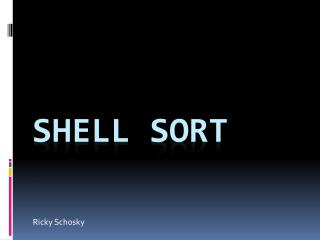DownloadDownload PresentationShell Sort

# Shell Sort

Download Presentation## Shell Sort

- - - - - - - - - - - - - - - - - - - - - - - - - - - E N D - - - - - - - - - - - - - - - - - - - - - - - - - - -
##### Presentation Transcript

1. Ricky Schosky Shell Sort

2. Purpose • Designed to be an improvement on insertion sort • Benefits: Adaptive, simple to implement • Runtime: O(n^3/2), however… • For the increment sequence, there are log3(n) increments, so the time complexity for nearly sorted data is O(n·log3(n)). • With larger sets of data…

3. General Algorithm • h = 1 • while h < n, • h = 3*h + 1 • while h > 0, • h = h / 3 for k = 1:h, insertion sort a[k:h:n]

4. My results • Smaller sample • -Insertion Sort Results- • Test 1: Comparisons = 2535 Swaps = 2438 • Test 2: Comparisons = 2668 Swaps = 2571 • Test 3: Comparisons = 2690 Swaps = 2596 • Test 4: Comparisons = 2469 Swaps = 2374 • Test 5: Comparisons = 2494 Swaps = 2398 • -Merge Sort Results- • Test 1: Comparisons = 547 Swaps = 586 • Test 2: Comparisons = 529 Swaps = 578 • Test 3: Comparisons = 541 Swaps = 599 • Test 4: Comparisons = 536 Swaps = 598 • Test 5: Comparisons = 544 Swaps = 604 • -Shell Sort Results- • Test 1: Comparisons = 503 Swaps = 376 • Test 2: Comparisons = 503 Swaps = 417 • Test 3: Comparisons = 503 Swaps = 356 • Test 4: Comparisons = 503 Swaps = 421 • Test 5: Comparisons = 503 Swaps = 408

5. My results • Larger sample-Insertion Sort Results- • Test 1: Comparisons = 24740876 Swaps = 24730890 • Test 2: Comparisons = 25057743 Swaps = 25047751 • Test 3: Comparisons = 25120119 Swaps = 25110126 • Test 4: Comparisons = 24955567 Swaps = 24945574 • Test 5: Comparisons = 24849112 Swaps = 24839123 • -Merge Sort Results- • Test 1: Comparisons = 120450 Swaps = 125931 • Test 2: Comparisons = 120465 Swaps = 125944 • Test 3: Comparisons = 120406 Swaps = 125931 • Test 4: Comparisons = 120444 Swaps = 125745 • Test 5: Comparisons = 120540 Swaps = 126065 • -Shell Sort Results- • Test 1: Comparisons = 120005 Swaps = 153630 • Test 2: Comparisons = 120005 Swaps = 150453 • Test 3: Comparisons = 120005 Swaps = 141641 • Test 4: Comparisons = 120005 Swaps = 151562 • Test 5: Comparisons = 120005 Swaps = 140806

6. Sources • http://www.sorting-algorithms.com/shell-sort • http://www.cs.auckland.ac.nz/software/AlgAnim/niemann/s_shl.htm • https://www.youtube.com/watch?v=qzXAVXddcPU